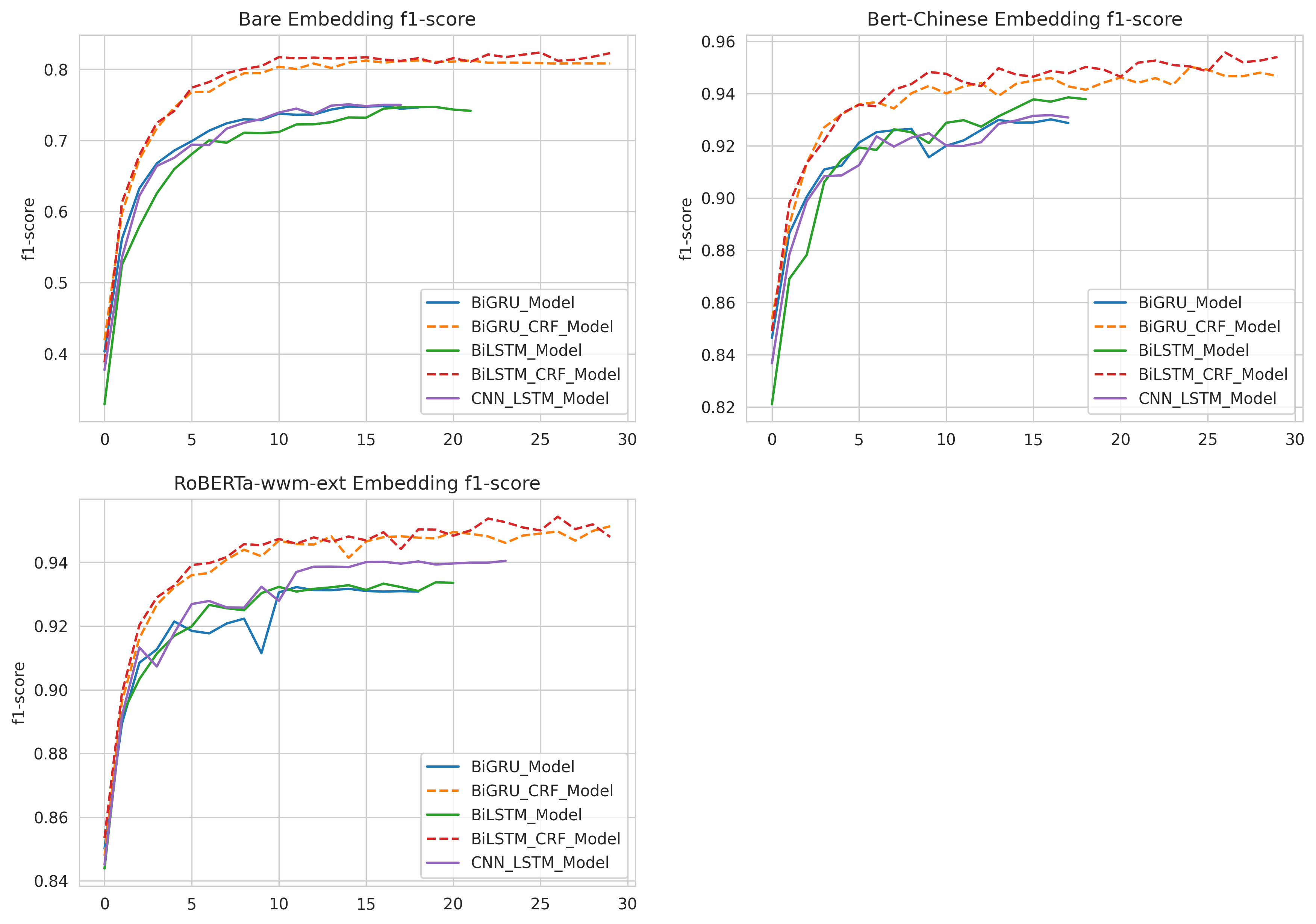Text Labeling Model¶

Kashgari provides several models for text labeling, All labeling models inherit from the BaseLabelingModel. You could easily switch from one model to another just by changing one line of code.

Name Info
CNN_LSTM_Model
BiLSTM_Model
BiGRU_Model

Train basic NER model¶

Kashgari provices basic NER corpus for expirement. You could also use your corpus in any language for training.

from kashgari.corpus import ChineseDailyNerCorpus

# Or use your own corpus
train_x = [['Hello', 'world'], ['Hello', 'Kashgari'], ['I', 'love', 'Beijing']]
train_y = [['O', 'O'], ['O', 'B-PER'], ['O', 'B-LOC']]

valid_x, valid_y = train_x, train_y
test_x, test_x = train_x, train_y

Or use your own corpus, it needs to be tokenized like this.

>>> print(train_x)
['海', '钓', '比', '赛', '地', '点', '在', '厦', '门', '与', '金', '门', '之', '间', '的', '海', '域', '。']

>>> print(train_y)
['O', 'O', 'O', 'O', 'O', 'O', 'O', 'B-LOC', 'I-LOC', 'O', 'B-LOC', 'I-LOC', 'O', 'O', 'O', 'O', 'O', 'O']

Then train our first model. All models provided some APIs, so you could use any labeling model here.

import kashgari

model = BiLSTM_Model()
model.fit(train_x, train_y, valid_x, valid_y)

# Evaluate the model

model.evaluate(test_x, test_y)

# Model data will save to `saved_ner_model` folder
model.save('saved_ner_model')

# To continue training, compile the newly loaded model first
model.fit(train_x, train_y, valid_x, valid_y)

That’s all your need to do. Easy right.

Sequence labeling with transfer learning¶

Kashgari provides varies Language model Embeddings for transfer learning. Here is the example for BERT Embedding.

from kashgari.embeddings import BertEmbedding

bert_embed = BertEmbedding('<PRE_TRAINED_BERT_MODEL_FOLDER>')
model = BiLSTM_Model(bert_embed, sequence_length=100)
model.fit(train_x, train_y, valid_x, valid_y)

You could easily change model’s hyper-parameters. For example, we change the lstm unit in BLSTMModel from 128 to 32.

hyper = BiLSTM_Model.default_hyper_parameters()
print(hyper)
# {'layer_blstm': {'units': 128, 'return_sequences': True}, 'layer_dropout': {'rate': 0.4}, 'layer_time_distributed': {}, 'layer_activation': {'activation': 'softmax'}}

hyper['layer_blstm']['units'] = 32

model = BiLSTM_Model(hyper_parameters=hyper)

Use custom optimizer¶

from kashgari.corpus import SMP2018ECDTCorpus
# Remember to import kashgari before than RAdam

model = BiLSTM_Model()
# This step will build token dict, label dict and model structure
model.build_model(train_x, train_y, valid_x, valid_y)
# Compile model with custom optimizer, you can also customize loss and metrics.
model.compile_model(optimizer=optimizer)

# Train model
model.fit(train_x, train_y, valid_x, valid_y)

Use callbacks¶

Kashgari is based on keras so that you could use all of the tf.keras callbacks directly with Kashgari model. For example, here is how to visualize training with tensorboard.

from tensorflow import keras
from kashgari.callbacks import EvalCallBack

model = BLSTMModel()

tf_board_callback = keras.callbacks.TensorBoard(log_dir='./logs', update_freq=1000)

# Build-in callback for print precision, recall and f1 at every epoch step
eval_callback = EvalCallBack(kash_model=model,
valid_x=valid_x,
valid_y=valid_y,
step=5)

model.fit(train_x,
train_y,
valid_x,
valid_y,
batch_size=100,
callbacks=[eval_callback, tf_board_callback])

It is very easy and straightforward to build your own customized model, just inherit the ABCLabelingModel and implement the default_hyper_parameters() function and build_model_arc() function.

from typing import Dict, Any

from tensorflow import keras

from kashgari.layers import L

import logging
logging.basicConfig(level='DEBUG')

class DoubleBLSTMModel(ABCLabelingModel):
"""Bidirectional LSTM Sequence Labeling Model"""

@classmethod
def default_hyper_parameters(cls) -> Dict[str, Dict[str, Any]]:
"""
Get hyper parameters of model
Returns:
hyper parameters dict
"""
return {
'layer_blstm1': {
'units': 128,
'return_sequences': True
},
'layer_blstm2': {
'units': 128,
'return_sequences': True
},
'layer_dropout': {
'rate': 0.4
},
'layer_time_distributed': {},
'layer_activation': {
'activation': 'softmax'
}
}

def build_model_arc(self):
"""
build model architectural
"""
output_dim = len(self.processor.label2idx)
config = self.hyper_parameters
embed_model = self.embedding.embed_model

layer_blstm1 = L.Bidirectional(L.LSTM(**config['layer_blstm1']),
name='layer_blstm1')
layer_blstm2 = L.Bidirectional(L.LSTM(**config['layer_blstm2']),
name='layer_blstm2')

layer_dropout = L.Dropout(**config['layer_dropout'],
name='layer_dropout')

layer_time_distributed = L.TimeDistributed(L.Dense(output_dim,
**config['layer_time_distributed']),
name='layer_time_distributed')
layer_activation = L.Activation(**config['layer_activation'])

# Define tensor flow
tensor = layer_blstm1(embed_model.output)
tensor = layer_blstm2(tensor)
tensor = layer_dropout(tensor)
tensor = layer_time_distributed(tensor)
output_tensor = layer_activation(tensor)

# Init model
self.tf_model = keras.Model(embed_model.inputs, output_tensor)

model = DoubleBLSTMModel()
model.fit(train_x, train_y, valid_x, valid_y)

Chinese NER Performance¶

We have run the classification tests on ChineseDailyNerCorpus. Here is the full code: colab link

• SEQUENCE_LENGTH = 100

• EPOCHS = 30

• EARL_STOPPING_PATIENCE = 10

• REDUCE_RL_PATIENCE = 5

• BATCH_SIZE = 64

Embedding Model Best F1-Score Best F1 @ epochs
0 RoBERTa-wwm-ext BiGRU_Model 93.22 11
1 RoBERTa-wwm-ext BiGRU_CRF_Model 95.13 29
2 RoBERTa-wwm-ext BiLSTM_Model 93.37 19
3 RoBERTa-wwm-ext BiLSTM_CRF_Model 95.43 26
4 RoBERTa-wwm-ext CNN_LSTM_Model 94.05 23
5 Bert-Chinese BiGRU_Model 93.01 16
6 Bert-Chinese BiGRU_CRF_Model 95.01 24
7 Bert-Chinese BiLSTM_Model 93.85 17
8 Bert-Chinese BiLSTM_CRF_Model 95.57 26
9 Bert-Chinese CNN_LSTM_Model 93.17 16
10 Bare BiGRU_Model 74.85 16
11 Bare BiGRU_CRF_Model 81.24 21
12 Bare BiLSTM_Model 74.7 19
13 Bare BiLSTM_CRF_Model 82.37 25
14 Bare CNN_LSTM_Model 75.07 14